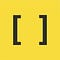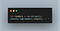# Array Helper Methods in ES6

## 12 methods that you can use to manipulate your data in a more creative way.Special signature(Square bracket)for defining Array in most of the programming language.

rray helper methods are the special kind of methods that work with data collection more easily and all the methods are themed around avoiding working with a manual for a loop. Array helper methods are more precisely used for data manipulation, which was introduced in ES6 or ECMAScript 6.

Before diving into the helper methods of an Array, let’s begin with the concept of an Array and Data Manipulation.

# So, What is Data Manipulation?

The prevalent thing is every day we are dealing with data & we have to manipulate that data in some way to perform some action and again return some sort of data. The process involves organizing data in some way, so, that data becomes more readable and understandable.

So, up to this, we got some basic idea of data manipulation. We only need to choose methods to manipulate data or we can say choose methods to perform an action. Now, let's understand what actually an Array is.

# What is an Array?

Let’s begin with an example of a variable holding some value in javascript.

`var number1 = 10;var number2 = 20;`

In this above example we are declaring a variable holding number type value i.e., 10 by number1 and 20 by number2. Now, what if there are thousands or millions of variables?

`var number1 = 10;var number2 = 20;var number3 = 30;........number10,000,000 = 98765434;`

We can easily declare thousands or millions of variables like in the above example. The problem with this type of declaration is the Complexity and Readability of code will be very large and poor. Here is where Array came into the picture.

The better approach is to use an array. An array is an indexed collection of a number of data elements. The main advantage of an array is we can represent multiple values by using a single variable name. You will get a clear understanding with an example.

`var arrayOfNumbers = [10,20,30,40,..........10_000_000];`

Now, Let’s start with the helper methods of an Array.

Since, the release of ES6 and forward, JavaScript has added great native support that makes dealing with array much easier. If we need to print the first 10 numbers on a console we most of the time use plain for loop to print numbers like:

This code simply sets a counter variable to 0, and prints the number each cycle through, adding 1 each time and completing at 9 since we say when variable i< 10 to break out. This can be done in a more convenient way using Array helper methods.

Ok, enough talking, Let’s start with our first helper method forEach().

# forEach()

array.forEach() calls a function on each element in an array.The iterator function deals with the operations that have to be performed. The array takes the parameter, which is the Iterator Function.

Syntax:

`array.forEach(function(currentValue, index, arr));`

forEach() Code Example:

Output:

`1,2,3,4,5,6,7,8,9,10`

# map()

array.map() loop through each item of the array, same as forEach() but map() returns the value of the array.map() calls the function for every element of the array in a particular order. Let’s start with a simple example:

`const arr = [{name:"bob",age:22}, {name:"jane",age:20}]let resArray = []for(let i = 0; i < arr.length; i++){    resArray.push(arr[i].name)}console.log(resArray);`

In this example, we are declaring an array containing key-value pairs as name and age. If our task is to get the values of a key in an array then we need some auxiliary space to store the final result i.e., bob, jane. We’ll start traversing through an array and on every key-value pair, we will get all the values of a key and push it into an auxiliary array. The output of the above code will be:

`[“James”,”Alice”]`

Now, let’s approach this using array.map() helper method.

Syntax:

`array.map(function(currentValue, index, arr));`

The following code shows the use of the map() helper method:

Output:

`[“James”,”Alice”]`

Easy? One of the biggest advantage to use Map over a for loop is that you don’t hold a temporary variable. map() significantly reduced the amount of code and avoid the use of a temporary variable.

# filter()

array.filter() contains a boolean condition.filter() returns an array consisting of all the elements that are true inside a function. It does not male any change in the original array. filter() is often used in filtering and sorting a list.

Syntax:

`array.filter(function(currentValue, index, arr));`

filter() code example:

Output:

`[{name:”banana”,color:”yellow”}]`

# find()

array.find() find value in the array (only the first value), if an element in the array satisfies the provided testing function else undefined if not found.It is really useful in a situation where we have a massive database and we have to find the ids of people.

find() code example:

output:

`{name:”jay”,age:1}`

# reduce()

array.reduce() takes two parameters.The first is the accumulator and the second is the initial value that we can set. Let’s understand this method with an example:

In this example, we are using reduce() helper method that takes two parameters accumulator and current value, and returns the sum of all elements in an array.

Output:

`15`

we can probably reimplement all the other helper methods by using reduce(). This is how reduce() works.

# some()

array.some() checks whether at least one element in the array passes the test implemented by the provided function. It returns a Boolean value. In some(), it is enough that one value will be matched with the condition and it will return true. Let’s take an example :

Output:

`true`

In this above example, some() will check if anyone element in an array matches the condition if it matches then the function will return true else false.

# every()

array.every() returns true if everything matches the condition. It returns a Boolean value. It is the same as the some() helper method but the only difference is it uses Logical AND instead of OR.

every() code example:

output:

`false`

# from()

array.from() is the method that creates a new instance of the `Array` from an array-like or iterable object.This method is a static method that is called using the Array class name.

Syntax :

`Array.from(arraylike, mapFunc, thisArg)`

In this Syntax :

• arrayLike — Array-like or iterable object to convert to an array.
• mapFunc(optional) — Map function that is called on each element.
• thisArg(optional)-Value to use as this when executing mapFunc.

Array.from() method of ES6 returns a new Array instance.

Array.from() code example :

Output :

`[ 1, 'A' ]`

# of()

Array.of() method always creates an array that contains the values that you pass to it regardless of the types or the number of arguments. The main difference between Array.of() and Array() constructor is that when we pass an integer value as a parameter in Array.of(), it creates an array with a single element that we pass whereas in Array() constructor it creates a length of a given size that is passed as a parameter.

Syntax :

`Array.of(element0[, element1[, ...[, elementN]]])`

Let’s understand with an example :Array() constructor creates an array of size 2.

Now let’s see Array.of() method example :

In this example, we passed the number 3 to the Array.of() method. The Array.of() method creates an array of one number.

# findIndex()

Array.findIndex() method returns the index of the first element in the array that satisfies the provided testing function. Otherwise, it returns `-1.`

Syntax :

`findIndex(testFn(element[, index[, array]])[, thisArg])`

Array.findIndex() code example :

The above example returns the index of the first occurrence of the number 7 in the numArray array.

# Keys()

Array.keys() method returns a new Array Iterator object that contains the keys for each index in the array. This method never ignores the empty string.

Syntax :

`arrayName.keys() `

In the above syntax arrayName is the name of your array.

Array.keys() example :

Output :

`01234`

# values()

Array.value() method returns a new Array object that contains the values for each index in the array.

Syntax :

`arrayName.values()`

In the above syntax arrayName is the name of your array.

Array.value() code example :

Output :

`abc`

# Conclusion

With that said, the majority of the time you can and should use these helper functions, but there may also be instances where the traditional for loop is a good solution as well.

Keep reading and Keep learning!!!

## The Startup

Get smarter at building your thing. Join The Startup’s +788K followers.

## Sign up for Top 10 Stories

### By The Startup

Get smarter at building your thing. Subscribe to receive The Startup's top 10 most read stories — delivered straight into your inbox, once a week. Take a look.

By signing up, you will create a Medium account if you don’t already have one. Review our Privacy Policy for more information about our privacy practices.

Check your inbox
Medium sent you an email at to complete your subscription.

## The Startup

Get smarter at building your thing. Follow to join The Startup’s +8 million monthly readers & +788K followers.

Written by

## Akshay Kumar

How I think as a Programmer and Logic that’s it.## The Startup

Get smarter at building your thing. Follow to join The Startup’s +8 million monthly readers & +788K followers.